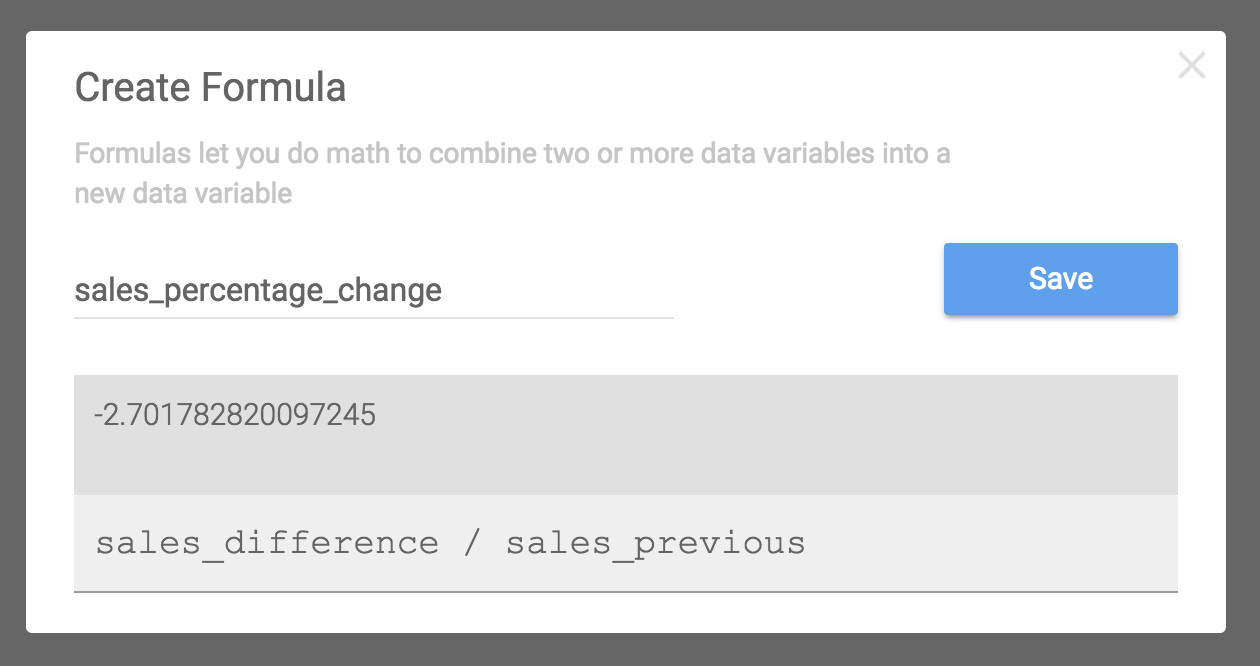## Why use formulas

Creating formulas in Wordsmith means that you can add some analysis to your data after uploading it. You can create formulas based on numbers, text, and any other data points you've uploaded to Wordsmith (column headers from your CSV file).

Here are some ways you may want to use formulas in Wordsmith:

• Averaging multiple data points (columns of your CSV)
• Calculating the percentage change between data
• Adding a static data point (like the current year)
• Putting different text strings together (like concatenate in Excel)

## How to use formulas

To add a Formula to your Project, start in the Template Editor and click the "Insert Data" button. You'll see a button on the top right of the data list called "Create Formula".

For example, in the Template below we say that home sales in San Diego have risen. But it might be interesting to say how much home sales have risen since last month. That's not a column from our data file, but we can create a formula to calculate how much sales have risen and add that to our sentence. Put your cursor anywhere in your Template where you want to insert a formula, click the "Insert Data" button, and then the "Create Formula" button.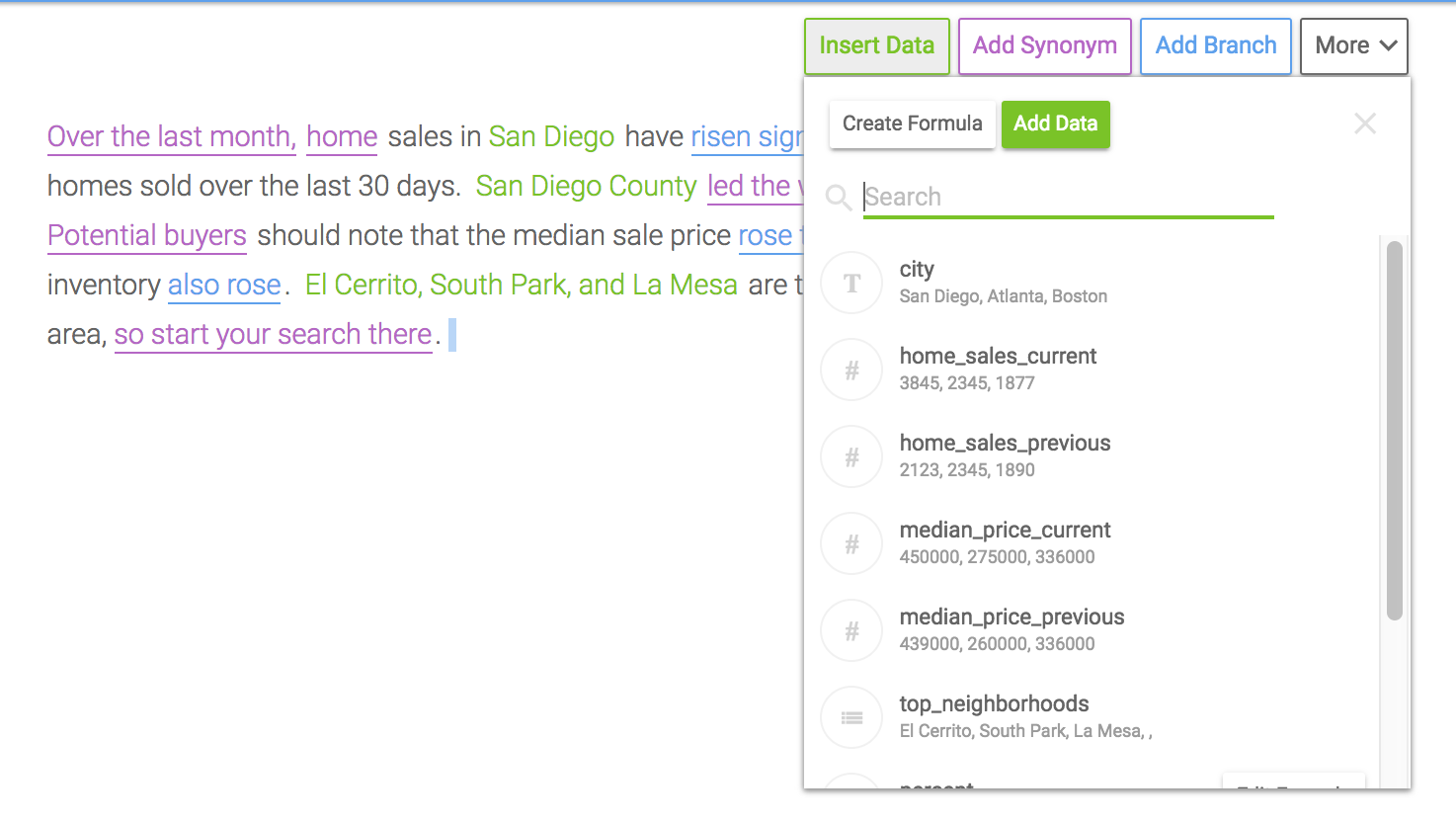The formula pane pops up. The first thing you should do is give your formula a name. Make sure it's one you'll remember later so that you can insert this data whenever you need to.

Then type in the formula you want to create. You can use the current data you've uploaded to this Project, numbers, or text strings. In this example, we've created a formula calculating the percentage change in home sales from the last time period.

You'll see the value of the formula you've created based on the first row of data from your CSV.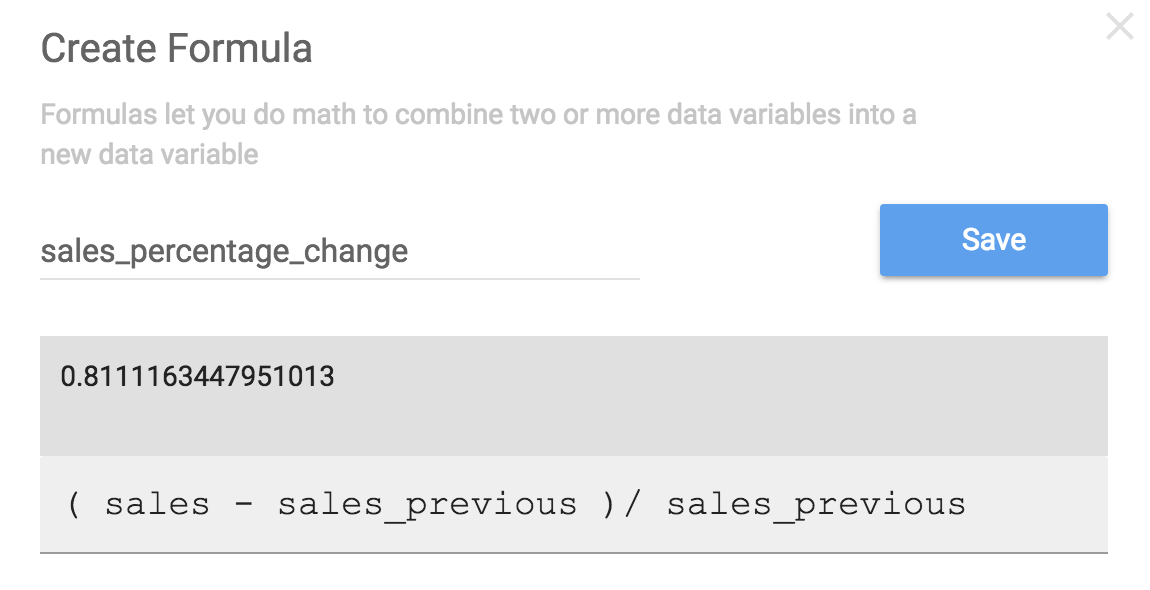Once you click the blue "Save" button, you'll see the formula you created inserted into your Template just like any other data, which you can then format however you like.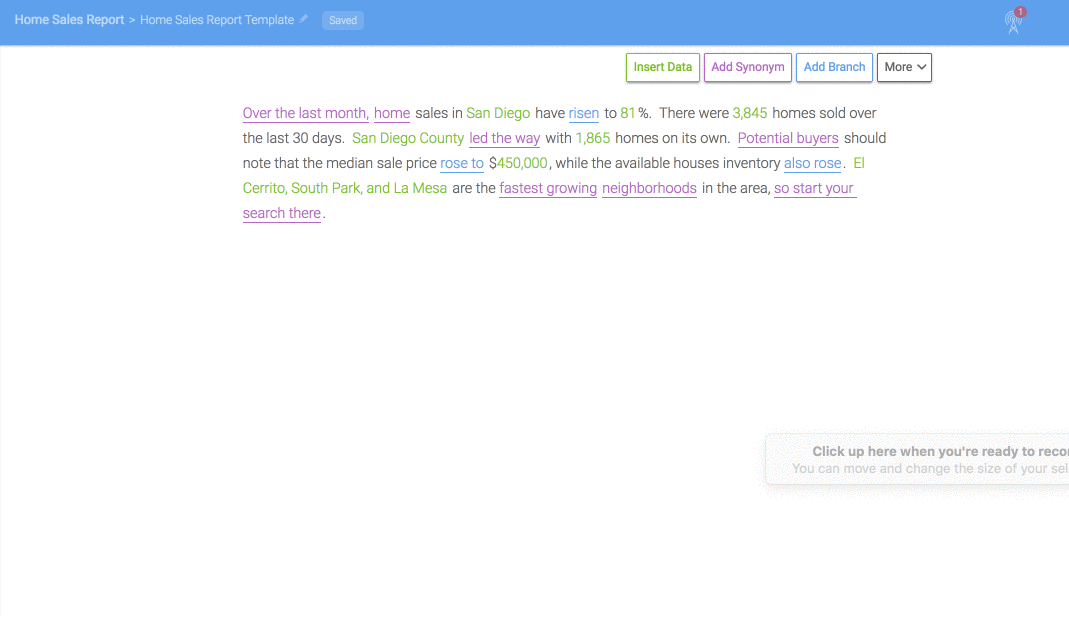❗️

Make sure your data isn't blank!

The Data Variables used in the formula cannot have blank values in the first 3 rows of the data you've uploaded to that Project. Wordsmith uses the first 3 data points to validate the formula, and so each one needs to have a value.

And you can now insert that formula in any other Template in this Project.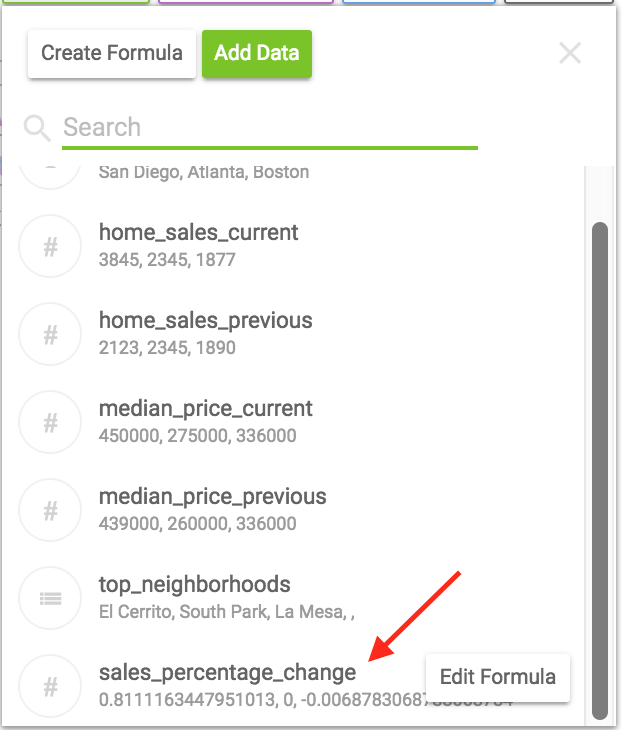You can also make any edits to it, or delete the formula if you no longer need it by clicking on the "Edit Formula" button in the data list. Delete will remove the formula wherever it has been inserted in your Template. To edit the formula, just make any changes and click "Update."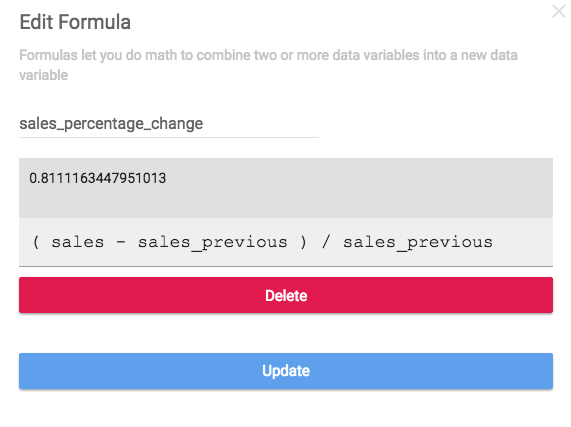You can use that formula in Branch rules. Maybe we want to say that sales have risen significantly if the sales percentage change is greater than 50%. Your formula will show up at the bottom of the data list when creating conditions and you can search for the name of it just like all other data.The formula you created can now be used across all other Templates within that Project.

🚧

You can even use that formula inside another formula! For example, if you wrote a formula for the difference between current sales and previous sales. You can then re-use that formula to calculate the percentage change between sales and previous sales.

## Different types of formulas

You can use formulas to create data values using other data you already have in your Project (like the example above where we created the percentage change from previous sales). Or you can create formulas with numbers (like an average) or string together text values. You can even create a formula with a static value, like the current year, which you can then reference in your Template and Branches.

Formulas using numbers
You can use numbers in Formulas, like creating an average of sales for the last 2 months.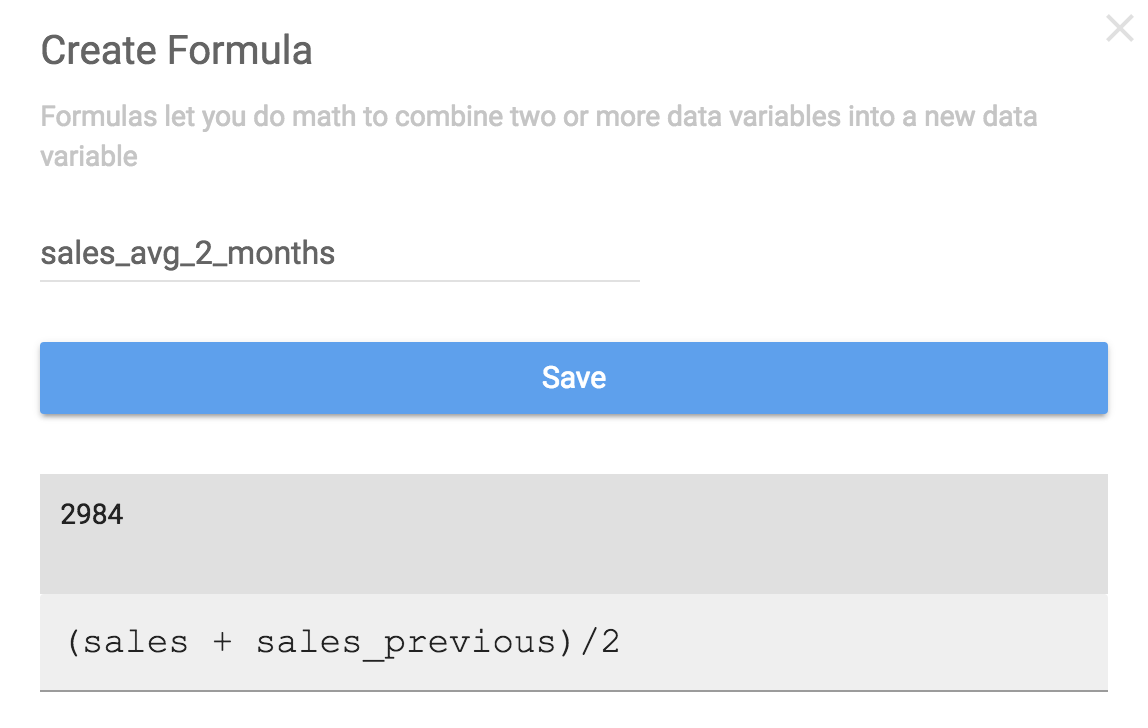Formulas with text
String together different text data. For example, if you have a sub-region and the larger region that it's in as data points in your CSV file, you could create a new data value that has both of them together.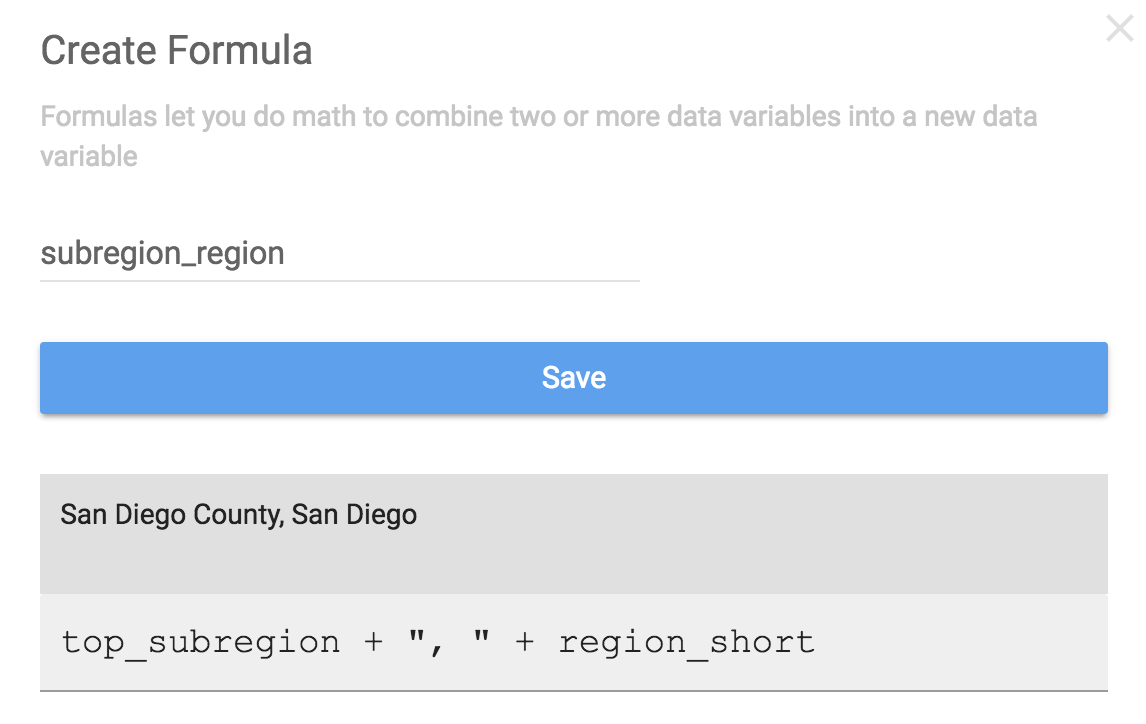Formulas for dates
Perform all of the same Get, Between, Ahead, and Ago functions in formulas that you can in your branch logic. Check out how to use these functions on the Data Types page.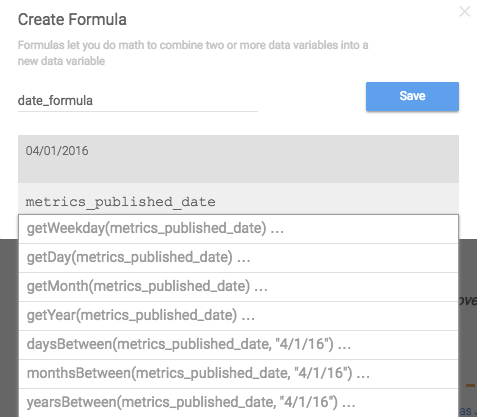Constant formulas
You don't have to include math or text operators to create a formula. You can just create a value that will not change. For example, maybe you want to reference the current year somewhere in your Template.## True/False Formulas

True/False, or boolean, statements can be used in formulas to create rules that you include in Branches throughout the Template. These are best used as a way to simplify a complicated Branch structure evaluating multiple data points or when you plan on re-using the same logic in more than one place.

In our home sales example, maybe we want to reference whether it's a seller's market in multiple Branches (if the median sales price is high and there has been significant growth in the price month over month). Instead of duplicating that logic in different Branches, we can write a formula for it once and insert it in multiple places.

And then we could use the formula in a Branch rule to write about the state of the housing market.

Which is simpler than having to write out the logic.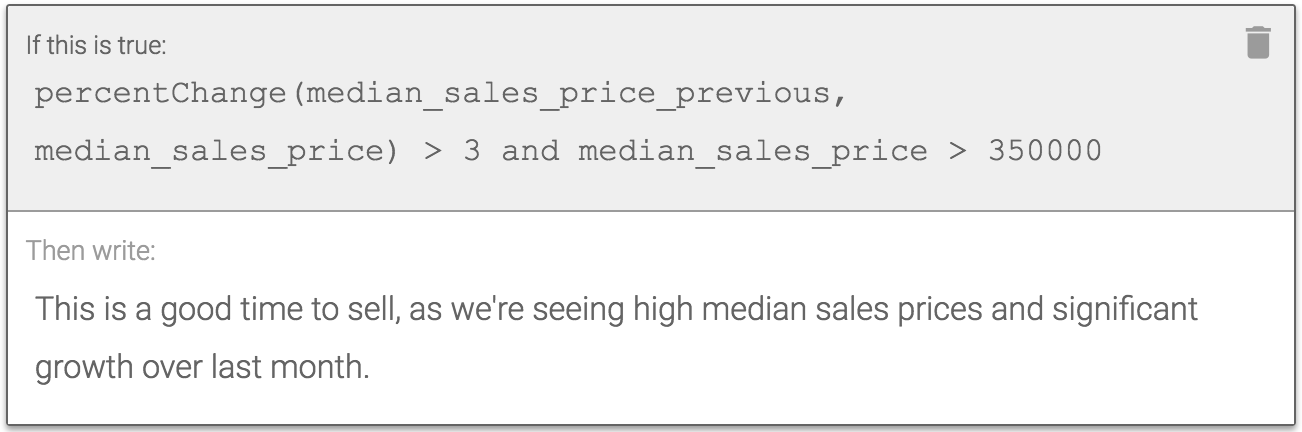And now we can just re-use this formula in another Branch without having to write it out again.

## Formulas referencing other formulas

You can include formulas you've already written in new formulas. You'll see all of the formulas you've created in the drop-down list along with the rest of your Data Variables.

For example, if you wanted to create a formula calculating the difference between current sales and previous sales, and a formula for the percentage change between current and previous. Those formulas can be built off each other.

Write the formula calculating the difference first: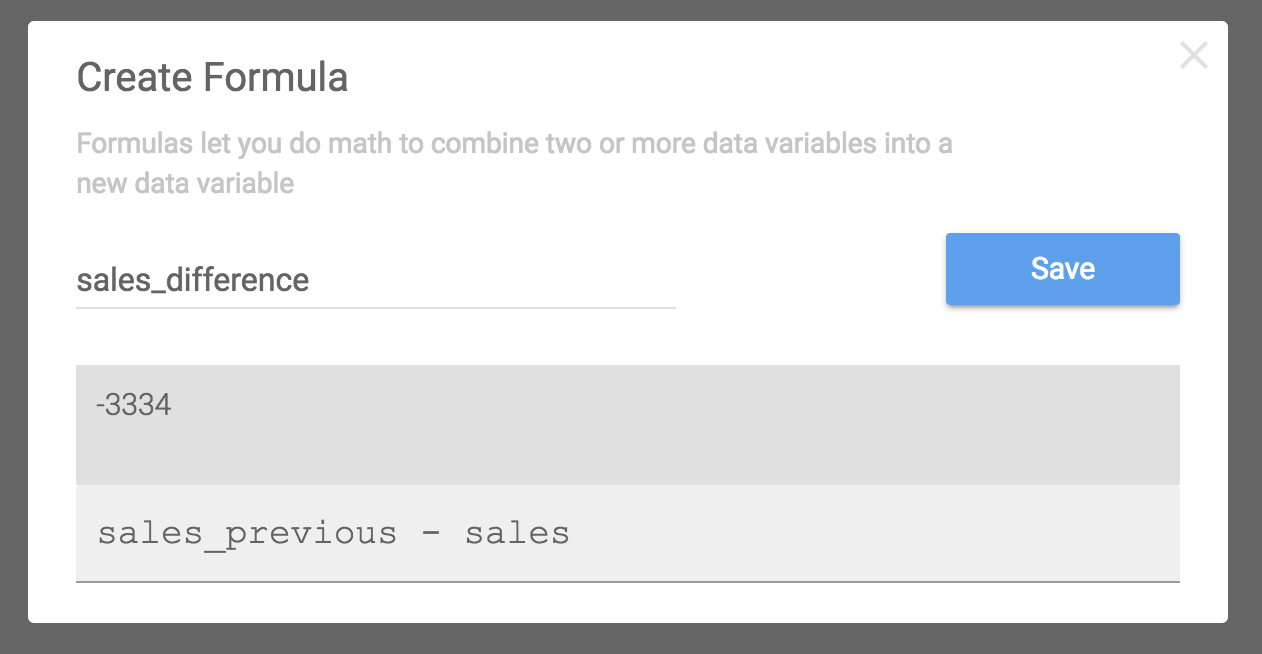Then you can use that formula to calculate the percentage change: